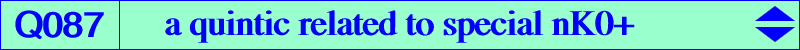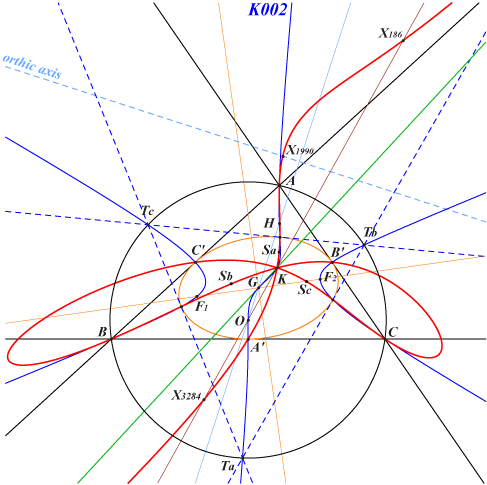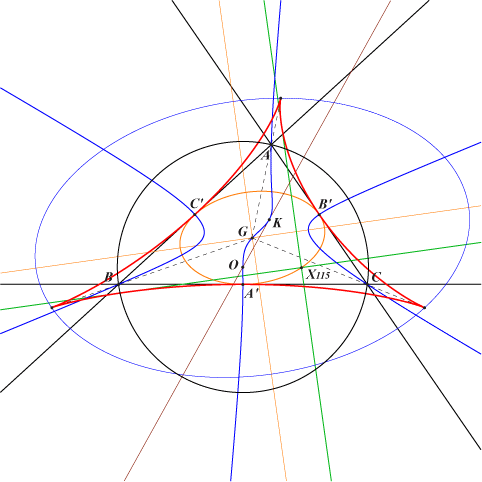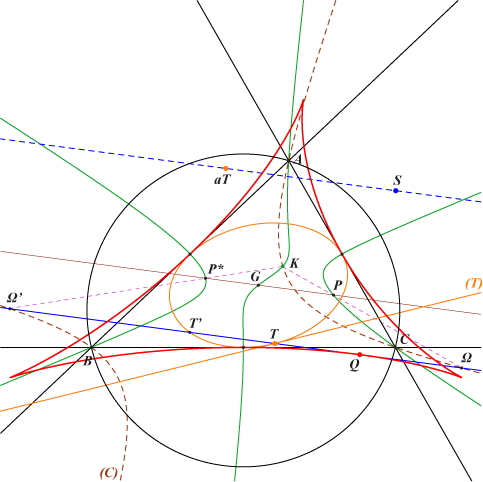too complicated to be written here. Click on the link to download a text file.X(6), X(44), X(187), X(524), X(1990), X(3284) Sa, Sb, Sc extraversions of X(44) midpoints of ABC other intersections of the Steiner inellipse and the Thomson cubic K002 more details belowFor any root P ≠ G on the Thomson cubic K002, there is a nK0 having a pencil of circular polar conics. Its pole Ω must the barycentric product of P and the infinite point of the line GP. In other words, for any nK0(Ω, P) of this kind, there is a line of points whose polar conics are circles which obviously form a pencil. This line is called the circular line of the cubic. When Ω is X(187), X(1990) we obtain K604, K393 respectively. The transformation P -> Ω maps the Thomson cubic to Q087. If P and P* are two isogonal conjugates on K002 (hence collinear with G), the corresponding poles Ω and Ω' have the following properties : K, P, Ω and K, P*, Ω' are collinear, Ω and Ω' lie on the trilinear polars of P and P* respectively, the lines GPP* and ΩΩ' are parallel, the points K, Ω, Ω' lie on a same circum-conic whose isogonal transform is a line through G meeting the line ΩΩ' on the Steiner inellipse, the enveloppe of ΩΩ' is a tricuspidal quartic tritangent to the sides of ABC at their midpoints, very similar to the Steiner deltoid. See below. Q087 passes through K which is a triple point with tangents passing through the points Ta, Tb, Tc of K002 on the circumcircle. Note that the tangents at A, B, C are the symmedians.This tricuspidal quartic is tritangent at the midpoints A', B', C' to the sidelines of ABC. The cusps are the images of A, B, C under the homothety with center G, ratio 3/2. The cuspidal tangents are the medians of ABC.Construction Let T be a point on the Steiner inellipse and (T) the tangent at this point. S is the isotomic conjugate of the infinite point of (T) and aT is the anticomplement of T. The parallel at G to the line S-aT meets the Thomson cubic at P, P*. The parallel at T to this same line contains Ω and Ω' which also lie on (C), the isogonal transform of the line TG. This latter parallel envelopes the quartic, the point of tangency Q being the reflection about T of its second intersection T' with the Steiner inellipse. The same construction applied to the reflection T1 of T about G gives another point Q1 on the quartic and the line QQ1 is also a tangent to the quartic.